# Repeating Character n Times in Excel

In daily life, we can use repeated characters to indicate the magnitude or priority of something. For example, a single “!” indicates a minor case, double “!!” indicates a medium case, and triple “!!!” indicates a high case, and so on. In an Excel worksheet, we can use REPT function to output a different number of repeated characters based on the input value to mark the difference. This approach is more convenient than creating a chart in Excel worksheet.

In today’s example, we want to indicate different incomes by outputting different numbers of “A” characters. Assuming the rule is one A per \$1000, for example, if income is \$1000, record an “A” in the “Level” column, and if income is 1750, just near 2000, but not enough for 2000, still an “A”.

Look at the following example. We use different amounts of repeated characters “A” to indicate different income levels.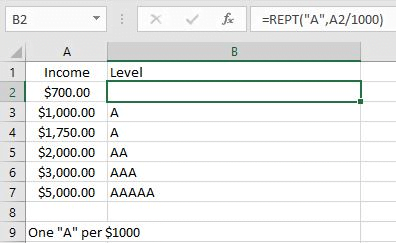In this example, you can also replace the character “A” with other characters, or even a string.

## FORMULA

Excel not only contains many very useful mathematical functions, but also contains several uncommon but useful functions. REPT function is one of these uncommon functions.

Excel REPT function can output repeating characters according to the input times. It is very helpful for us to build a simple comparison with normal or special characters.

Syntax:

`= REPT(text, number_times)`

Example:

`REPT(“*”,5) – Outputs *****`

In this example, the text is the letter “A”, character “A” should be enclosed into double quotes, and the number_times is A2/1000, depending on how many 1000s the income value contains.

The formula is:

`= REPT(“A”, A2/1000)`

## EXPLANATION

REPT is a very simple function in Excel. We only need to confirm the output character and the number of times to output proper characters.

### 1.number_times Is Not an Integer & <1

In this example, the number of outputs is determined by the mathematical expression A2/1000.

A2/1000 -> Calculate repeating times

Calculate A2/1000 in Excel formula bar. The result is 0.7.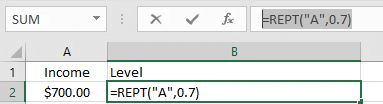The REPT function now has two parameters confirmed, the output character is “A” and the times is “0.7”. Since times is not an integer and is less than 1, it will be truncated, so the parameter is equal to 0, no “A” is output, and an empty cell is displayed in B2.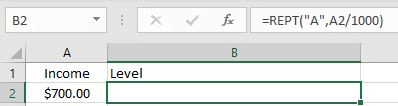### 2.number_times Is Not an Integer & >1

In B4, the formula is:

`=REPT("A",A4/1000).`

A4/1000=1.5 –> Repeating times 1.5 is not an integer, decimal part is truncated.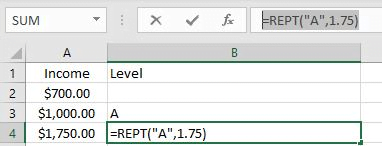1.5 is rounded down to the nearest integer 1. So only one “A” is output and displayed in B4.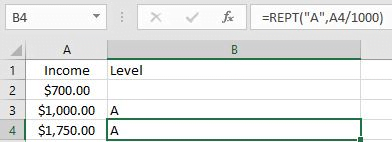### 3.number_times Is an Integer

In B3, the formula is =REPT(“A”,A3/1000).

A3/1000=1 –> Repeating times is an integer

So REPT function only returns one “A”.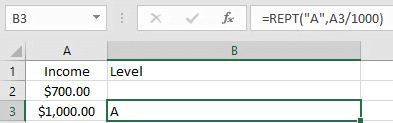## EXPAND

Wingdings character set and equivalent Unicode characters (alanwood.net)

Sometimes we may want to display some special characters or icons in an Excel worksheet. We can still apply the REPT function to output characters, but these special characters cannot be entered into Excel formulas because they are not accepted. At this point we need to get help from CHAR function in Excel.

CHAR function can return a character based on the entered number.

Syntax: CHAR(Number)

Depending on the font, CHAR will return different characters even if the same number is entered.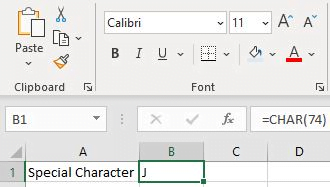Pick font Calibri.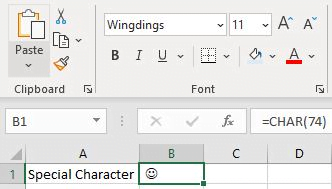Pick font Wingdings.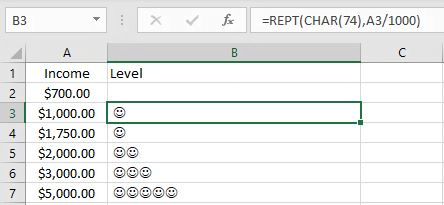To output the smileface instead of letter “A” in the above example, we simply enter char(74) in the REPT “text” argument to replace letter “A”.

### Related Functions

• Excel CHAR function
The Excel CHAR function returns the character specified by a number (ASCII Value).The CHAR function is a build-in function in Microsoft Excel and it is categorized as a Text Function. The syntax of the CHAR function is as below:=CHAR(number)….
• Excel REPT function
The Excel REPT function repeats a text string a specified number of times.The REPT function is a build-in function in Microsoft Excel and it is categorized as a Text Function.The syntax of the REPT function is as below:= REPT  (text, number_times)….
Related Posts

Calculate Cumulative Loan Interest in Excel

What is Cumulative Loan Interest? When comparing different types of loans, many people want to know what is Cumulative Loan Interest. The sum of all interest payments you've made on a loan is referred to as cumulative interest. Different lenders ...

Calculate Cumulative Loan Principal Payments in Excel

How do you Calculate Cumulative Loan Principal Payments in Excel? There are a few different ways to calculate this information, but one way has been proven to be accurate more often than others. One option is to use the CUMPRINC ...

Trap Error or Replace Error by Specific Value with IFERROR function

We often use Excel formulas in our work life, and we may encounter this situation that the formula throws an error and finally an error like #DIV/0! Is displayed in the cell. In today’s tutorial, we will introduce you the ...

Calculate Compound Interest in Excel

This article will show you how to calculate compound interest in Excel. It will help you calculate the interest you will accrue on a given amount of money. There are several ways to calculate compound interest. One of the simplest ...

Count Attendance and Absence with COUNTIF function

In our campus life and work life, we usually record everyone's attendance. Today we will introduce you the application of Excel COUNTIF function to count the attendance. Using a week as an example, we use the following example to show ...

Find the Closest Data to the Data Provided in Excel

In our daily work, we may encounter such an issue that to find the closest value to a certain value. In fact, Excel internal functions can help us solve this problem. In today’s article, we will show you how to ...

Average Of Numbers With Multiple Criteria In Excel

Have you ever come across a task to calculate the average of the numbers with respect to multiple criteria? Are you tired of doing this cumbersome task manually? Are you willing to do this task smartly in just a matter ...

Calculate Average Of Last 5 Or N Values In Columns

Suppose you come across a task where you need to calculate the average of the last 2 or 3 numeric values, then what would you do? If you are new to Excel, then your first attempt might be doing this ...

Calculating Average Of The Numbers

Are you weary of investing a lot of time and effort in manually calculating the average of the numbers by including or excluding 0 and calculating the average of the top 3 scores? Then congratulations because you have just landed ...

Extract Multiple Lines From A Cell

Suppose that you have listed some text in a single cell which is separated by the line break(you can do it by pressing ALT + ENTER after entering the text), and now you want to extract multiple lines of text ...

Sidebar